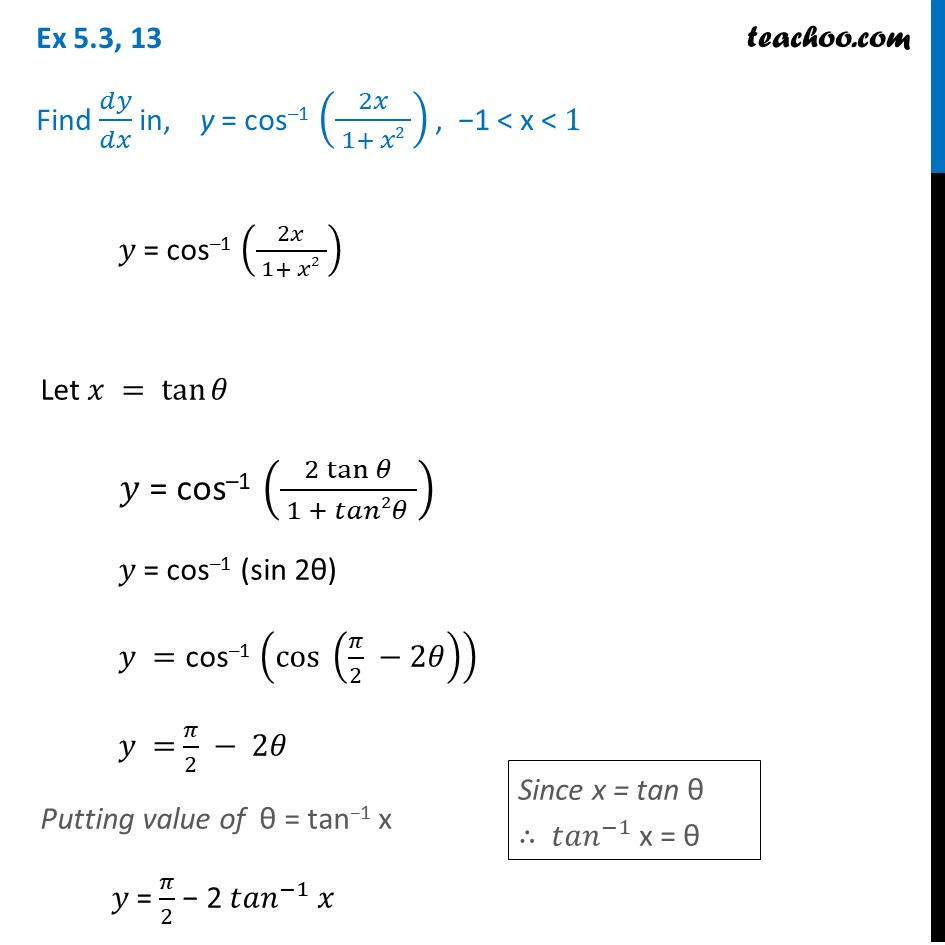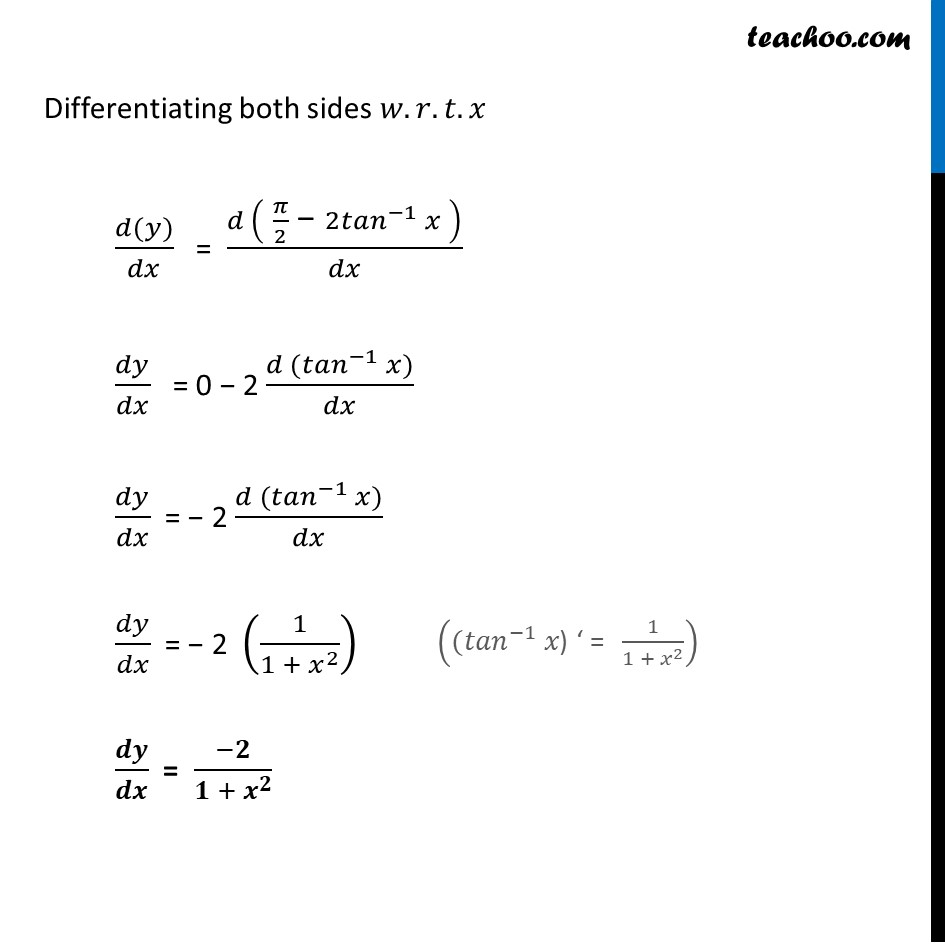Ex 5.3

Chapter 5 Class 12 Continuity and Differentiability
Serial order wiseLearn in your speed, with individual attention - Teachoo Maths 1-on-1 Class

### Transcript

Ex 5.3, 13 Find 𝑑𝑦/𝑑𝑥 in, y = cos–1 (2𝑥/( 1+ 𝑥2 )) , −1 < x < 1 𝑦 = cos–1 (2𝑥/( 1+ 𝑥2 )) Let 𝑥 = tan⁡𝜃 𝑦 = cos–1 ((2 tan⁡𝜃)/( 1 + 𝑡𝑎𝑛2𝜃 )) 𝑦 = cos–1 (sin 2θ) 𝑦 ="cos–1" (〖cos 〗⁡(𝜋/2 −2𝜃) ) 𝑦 = 𝜋/2 − 2𝜃 Putting value of θ = tan−1 x 𝑦 = 𝜋/2 − 2 〖𝑡𝑎𝑛〗^(−1) 𝑥 Since x = tan θ ∴ 〖𝑡𝑎𝑛〗^(−1) x = θ Differentiating both sides 𝑤.𝑟.𝑡.𝑥 (𝑑(𝑦))/𝑑𝑥 = (𝑑 (" " 𝜋/2 " − " 〖2𝑡𝑎𝑛〗^(−1) 𝑥" " ))/𝑑𝑥 𝑑𝑦/𝑑𝑥 = 0 − 2 (𝑑〖 (𝑡𝑎𝑛〗^(−1) 𝑥))/𝑑𝑥 𝑑𝑦/𝑑𝑥 = − 2 (𝑑〖 (𝑡𝑎𝑛〗^(−1) 𝑥))/𝑑𝑥 𝑑𝑦/𝑑𝑥 = − 2 (1/(1 + 𝑥^2 )) 𝒅𝒚/𝒅𝒙 = (−𝟐)/(𝟏 + 𝒙^𝟐 ) ((〖𝑡𝑎𝑛〗^(−1) 𝑥") ‘ = " 1/(1 + 𝑥^2 ))